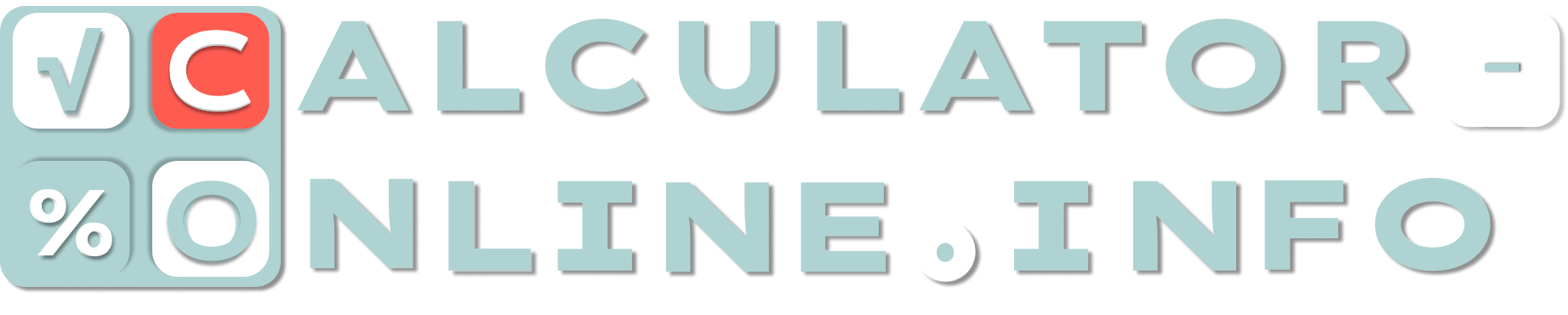# Calculator of human proportions — Know your body proportion

Ideal body proportion is not only about bust, hips and waist. Here is body proportion calculator which determines the coefficient of norm by such parameters as circumference of neck, upper arm, hip and lowers leg.

Ideal body proportion calculator for bodybuilders needs some other measurements. You can also find in on our website.

## What is your body proportion?

Body proportion measurements are carried out using a soft ruler. The ruler should be taut, but at the same time not pinch your body. It allows you to measure yourself correctly.

What you should measure for male & female body proportions calculator:

• circumference of neck;
• upper arm circumference;
• hip circumference;
• lower leg circumference.

After that you should write down all the measurements for body proportion calculation and put on the bottom “Calculate”.

## What is a good body proportion?

Due to this body proportions calculator the norm depends on the gender:

• Coefficient from 54 to 62 is good for women.
• Coefficient from 46 to 52 is good for men.

Compare the result of human proportions calculator with these norms to evaluate your body.

Rating
( 3 assessment, average 5 from 5 )Mathematician/ author of the article